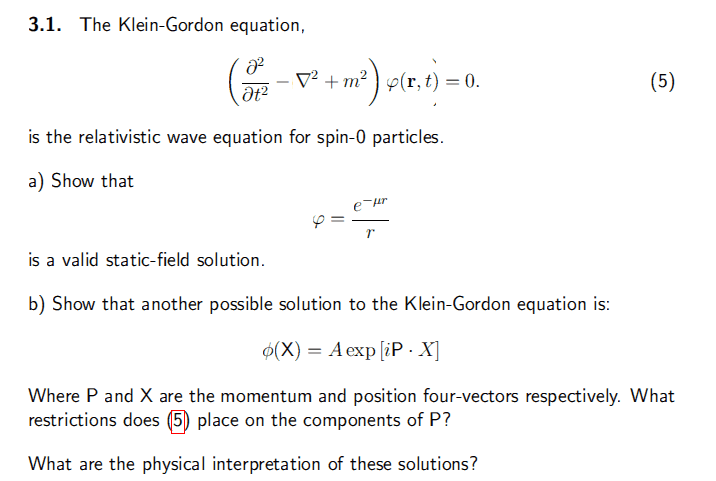# Yukawa potential and the Klein Gordon

## Homework Statement

a)Show that the yukawa potential is a valid static-field euation
b)Show this solution also works## The Attempt at a Solution

Part (a)

Using the relation given, I got

$$LHS = \frac{e^{-\mu r}}{r} \left[ (m^2 - \mu^2) - \frac{2\mu}{r} - \frac{2}{r^2} \right]$$

So for LHS = RHS = 0, it means that ##m = \mu = -\frac{1}{r}##, so the wavefunction is ##\phi = \frac{e}{r}##.

What does this mean?

Part (b)

Using the relation given, I got

$$LHS = \left( E^2 - p^2 - m^2 \right)\phi$$

For LHS = RHS = 0, it means that ## E^2 - p^2 = m^2##.

Does this mean that the particle must be on the mass shell? i.e. an external particle and not an internal (virtual) particle?

What are the physical interpretations of these?